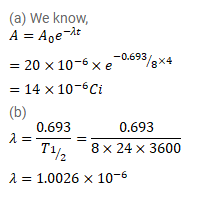# Radioactive 131I has a half-life of 8.0 days.

Question:

Radioactive ${ }^{131}$ I has a half-life of $8.0$ days. A sample containing ${ }^{131}$ I has activity $20 \mu$ Ci at $t=0$.

(a) What is its activity at $t=4.0$ days?

(b) What is its decay constant at $t=4.0$ days?

Solution: